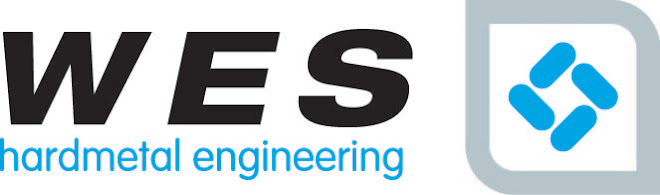## Wednesday, 1 June 2011

### Does Tungsten Carbide have much elasticity?

Young's Modulus of Elasticity

Young's Modulus of Elasticity is an indication of the elasticity or bendability of Tungsten Carbide. Bendability increases with the increase in binder percentage. So carbide with a Cobalt binder percentage of 3% will be less elastic that one with a binder percentage of 6%.

Poisson's Ratio

Poisson's ratio is the ratio of transverse contraction strain to longitudinal extension strain in the direction of stretching force.
Poisson’s ratio is the relation of the strain transverse to the length of the tension sample and the longitudinal strain. It is a calculation of the transverse contraction of the sample in reaction to the tensile loading. If a section of homogeneous material is subjected to a tensile load and increases in length by a portion, it will exhibit a reduction in dimensions widthways. If the bar is maintained within the elastic limits of the material, the ratio of the strains will be a constant.The compressive side of Poisson's ratio may most easily be described by thinking of a marshmallow held between two flat plates. As the plates are pushed together the marshmallow is compressed and squashes out. All metals, even Tungsten Carbide, squash out at least a very small amount. The ratio varies only slightly with the amount of cobalt binder.

The Poisson’s ratio of Tungsten Carbide is around 0.24, compared to Titanium which is 0.33.

Mean Coefficient of Thermal Expansion

This indicates the amount of expansion which might be expected when heat is applied to the material. The expansion rate increases with temperature increase. The more binder present the higher the expansion rate. Tungsten Carbide is about 1/3 to 1/2 that of tool steel.

1.Really a Valuable blog the Tool and Tungsten Carbide Blades.
Thanks for sharing a nice blog, this will really useful who want to detailed information in the like another machinery Tool.
2.